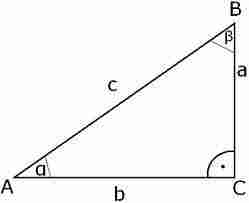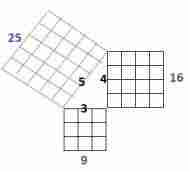# Pythagoras Theorem Calculator with examples

Please select the side you wants to calculate and enter the value of other two sides.

 Select side Select Hypotenuse side (c) Adjacent side (a) Opposite side (b)

 Adjacent side (a): Opposite side (b): Hypotenuse side (c):

In a right angled triangle: the square of the hypotenuse is equal to the sum of the squares of the other two sides.

Suppose right triangle with sides a and b and hypotenuse c then c2 = a2 + b2

Or c = √(a2 + b2)

Or a = √(c2 - b2)

Or b = √(c2 + a2)## Examples :

1. Find the hypotenuse where two sides a = 6 cm and b = 8 cm.

The formula for hypotenuse c = √(a2 + b2)

= √(62 + 82)

= √(6X6 + 8x8)

= √(36 + 64)

= √(100)

=10 cm

2. Find the hypotenuse where two sides a = 4 and b = 3.

The formula for hypotenuse c = √(a2 + b2)

= √(42 + 32)

= √(4X4 + 3x3)

= √(16 + 9)

= √(25)

> =5